### Home > INT3 > Chapter 2 > Lesson 2.2.1 > Problem2-44

2-44.

Write a possible equation for each of these graphs. Assume that all axes are scaled by one unit. Check your equations on a graphing calculator.

1.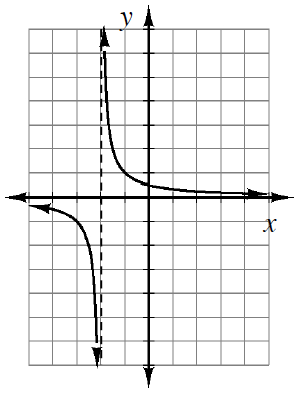Notice that this is a hyperbola $(y\ =\frac{1}{x})$.

1.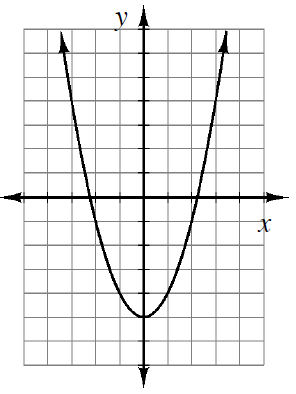Notice that the vertex is $(0,−5)$.

1.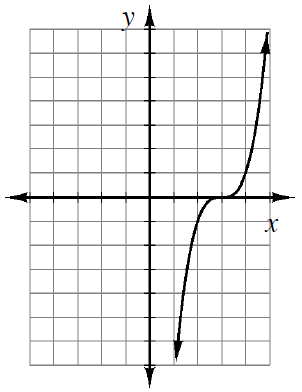Notice that this is a cubic graph.

1.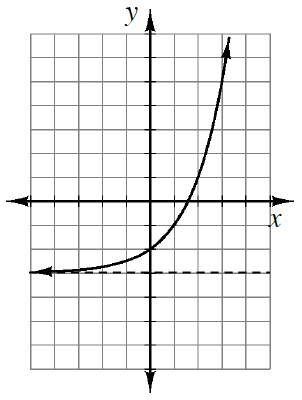Notice that this is an exponential graph.

1.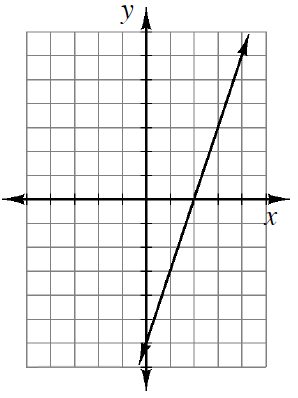Notice that the $y$-intercept is $(0,−6)$.

1.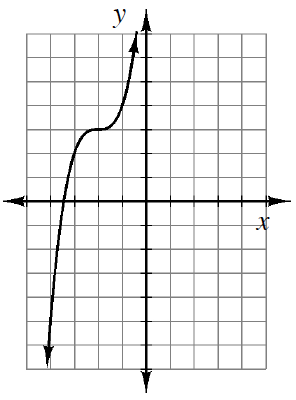See the hints from parts (a)-(e) to help you solve parts (f)-(i).

1.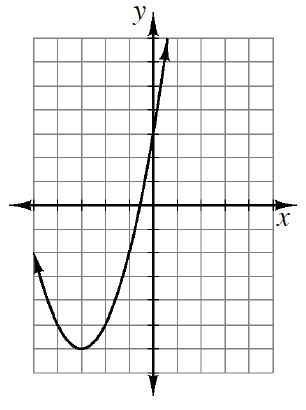1.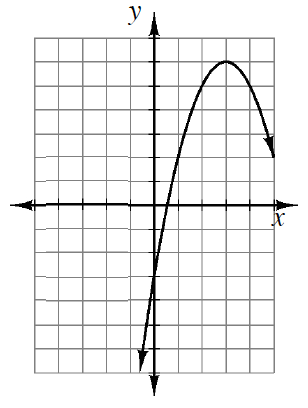1.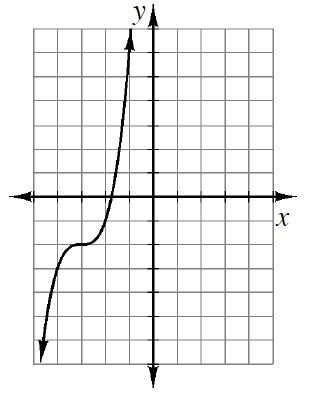Use the eTool below to graph the equations.
Click the link at right for the full version of the eTool: Int3 2-44 HW eTool